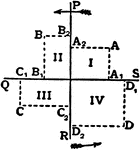This mathematics ClipArt gallery offers 7 illustrations of descriptive geometry, which is defined as geometric principles displayed on an image and explaining the method or technique used to solve the problem.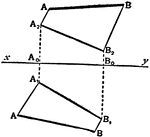### Point Distance

To determine the distance between two points A, B given by their projections.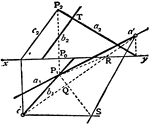### Angle of Two Lines

To find the angle between two given lines a, b of which the projections a1, b1 and a2, b2 are given.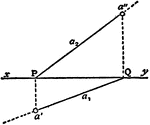### Line Projection

To find the projections of a line which joins two points, A, B given by their projections.### Parallel Planes

To draw a plane through a given point parallel to a given plane.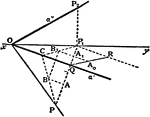### Angle of Two Planes

To determine the angle between two planes.### Draw Polygon

Draw the plan and elevation of a polygon of which the real shape and position in a given plane are known.